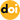### Analysis of the Charging Characteristics of High Voltage Capacitor Chargers Considering the Transformer Stray Capacitance

Vol. 13, No. 3, pp. 329-338, May  201310.6113/JPE.2013.13.3.329

###### Abstract

In this paper, the charging characteristics of series resonant type high voltage capacitor chargers considering the transformer stray capacitance have been studied. The principles of operation for the four operational modes and the mode changes for the four different switching frequency sections are explained and analyzed in the range of switching frequency below the resonant frequency. It is confirmed that the average charging currents derived from the above analysis results have non-linear characteristics in each of the four modes. The resonant current, resonant voltage, charging current, and charging time of this capacitor charger as variations of the switching frequency, series parallel capacitance ratio (k=Cp/Cs), and output voltage are calculated. From the calculation results, the advantages and disadvantages arising from the parallel connection of this stray capacitance are described. Some methods to minimize charging time of this capacitor charger are suggested. In addition, the results of a comparative test using two transformers whose stray capacitances are different are described. A 1.8 kJ/s prototype capacitor charger is assembled with a TI28335 DSP controller and a 40 kJ, 7 kV capacitor. The analysis results are verified by the experiment.

###### Statistics
Show / Hide Statistics

Cumulative Counts from September 30th, 2019
Multiple requests among the same browser session are counted as one view. If you mouse over a chart, the values of data points will be shown.